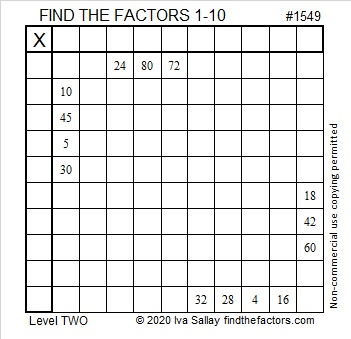# 1549 and Level 2

### Today’s Puzzle:

Write the numbers from 1 to 10 in both the first column and the top row so that the given clues are the products of the numbers you write.### Factors of 1549:

• 1549 is a prime number.
• Prime factorization: 1549 is prime.
• 1549 has no exponents greater than 1 in its prime factorization, so √1549 cannot be simplified.
• The exponent in the prime factorization is 1. Adding one to that exponent we get (1 + 1) = 2. Therefore 1549 has exactly 2 factors.
• The factors of 1549 are outlined with their factor pair partners in the graphic below.How do we know that 1549 is a prime number? If 1549 were not a prime number, then it would be divisible by at least one prime number less than or equal to √1549. Since 1549 cannot be divided evenly by 2, 3, 5, 7, 11, 13, 17, 19, 23, 29, 31, or 37, we know that 1549 is a prime number.

### More about the number 1549:

1549 is the sum of two squares:
35² + 18² = 1549

1549 is the hypotenuse of a Pythagorean triple:
901-1260-1549, calculated from 35² – 18², 2(35)(18), 35² + 18².

Here’s another way we know that 1549 is a prime number: Since its last two digits divided by 4 leave a remainder of 1, and 35² + 18² = 1549 with 35 and 18 having no common prime factors, 1549 will be prime unless it is divisible by a prime number Pythagorean triple hypotenuse less than or equal to √1549. Since 1549 is not divisible by 5, 13, 17, 29, or 37, we know that 1549 is a prime number.